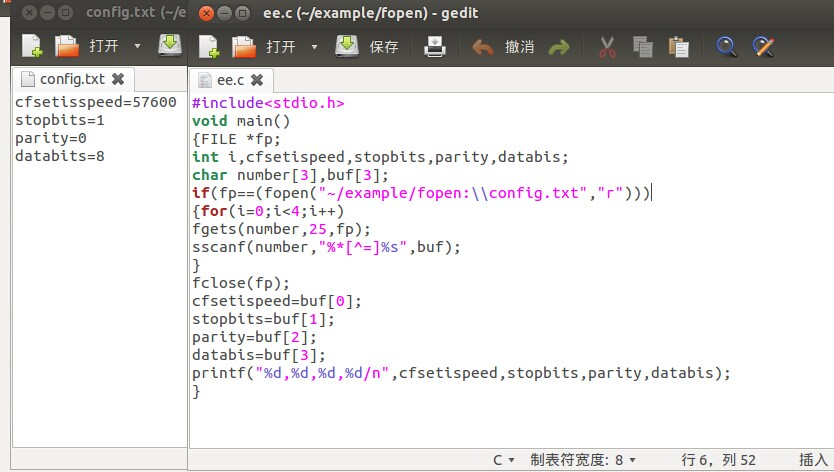# 请大家帮忙看看，我自己编译了还是没成功？！

2023-03-15 16:12:56

cfsetispeed=57600
stopbits=1
parity=0
databits=8## 2 回答TA贡献1626条经验 获得超8个赞

#include<stdio.h>
void main()
{
FILE *fp = NULL;
int number = {};

if (fp = fopen("e:\\1.txt", "r"))
{
for (int i = 0; i < 4; i++)
fscanf(fp, "%*[^=]=%d", number+i);

fclose(fp);
printf("%d, %d, %d, %d\n", number, number, number, number);
}
return;
}

..................................................................TA贡献1726条经验 获得超3个赞

fopen函数用来打开一个文件，其调用的一般形式为： 文件指针名=fopen(文件名，使用文件方式) 其中，“文件指针名”必须是被说明为FILE 类型的指针变量，“文件名”是被打开文件的文件名。 “使用文件方式”是指文件的类型和操作要求。“文件名”是字符串常量或字符串数组。

·字符读写函数 ：fgetc和fputc
·字符串读写函数：fgets和fputs
·数据块读写函数：freed和fwrite
·格式化读写函数：fscanf和fprinf

C语言源程序如下所示：

#include <stdio.h>
#include <stdlib.h>
#include <assert.h>
typedef struct student{
char name;
int no;
char sex;
float score;
} stu;

int main(int argc, char* argv[])
{
//打开文件
FILE * r=fopen("A.txt","r");
assert(r!=NULL);
FILE * w=fopen("B.txt","w");
assert(w!=NULL);

//读写文件
stu a;
int i=0;
while(fscanf(r,"%s%d%s%f",a[i].name,&a[i].no,a[i].sex,&a[i].score)!=EOF)
{
printf("%s\t%d\t%s\t%g\n",a[i].name,a[i].no,a[i].sex,a[i].score);//输出到显示器屏幕
fprintf(w,"%s\t%d\t%s\t%g\n",a[i].name,a[i].no,a[i].sex,a[i].score);//输出到文件B.txt
i++;
}

//关闭文件
fclose(r);
fclose(w);

system("pause");
return 0;
}

• 2 回答
• 0 关注
• 7 浏览

0/150